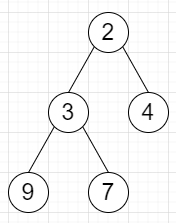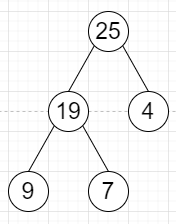# Program to find a tree by updating values with left and right subtree sum with itself in Python

Suppose we have a binary tree, we have to find the same tree but every node's value is replaced by its its value + all of the sums of its left and right subtrees.

So, if the input is likethen the output will beTo solve this, we will follow these steps −

• Define a function tree_sum() . This will take root of a tree

• if root is null, then

• return 0

• data of root := tree_sum(left of root) + tree_sum(right of root) + data of root

• return data of root

• From the main method, do the following:

• tree_sum(root)

• return root

Let us see the following implementation to get better understanding −

## Example

class TreeNode:
def __init__(self, data, left = None, right = None):
self.data = data
self.left = left
self.right = right

def inorder(root):
if root:
inorder(root.left)
print(root.data, end = ', ')
inorder(root.right)

class Solution:
def solve(self, root):
def tree_sum(root: TreeNode):
if root is None:
return 0
root.data = tree_sum(root.left) + tree_sum(root.right) + root.data
return root.data
tree_sum(root)
return root
ob = Solution()
root = TreeNode(2)
root.left = TreeNode(3)
root.right = TreeNode(4)
root.left.left = TreeNode(9)
root.left.right = TreeNode(7)
ob.solve(root)
inorder(root)

## Input

root = TreeNode(12)
root.left = TreeNode(8)
root.right = TreeNode(15)
root.left.left = TreeNode(3)
root.left.right = TreeNode(10)

## Output

9, 19, 7, 25, 4,